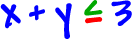Ok, now you're an expert at graphing lines likeBut, what if we stick an inequality in there?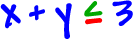Let's graph the line: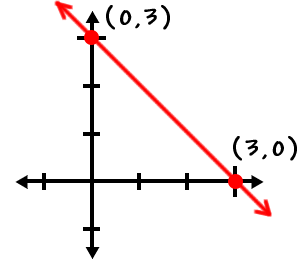This takes care of the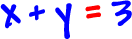part ... So, what about the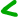?

Remember that this rectangular coordinate grid is just made up of a bunch of ( x , y ) points.

All the points ON the line work in this part:Now, we just need to find all the points that work in this part: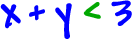Here's how we find these points:

We just pick a point OFF the line and test it!The origin, ( 0 , 0 ), is really easy to work with, so let's try it:( 0 , 0 ) works in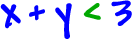!

Here's the deal:

If ( 0 , 0 ) works, then ALL the points
on the same side of the line will work.

Don't believe me?  Let's try a couple more: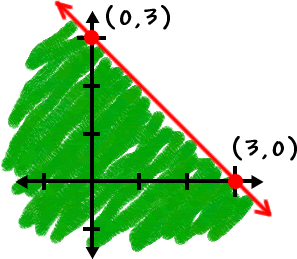All the ( x , y ) points on the line and in the shaded region work in